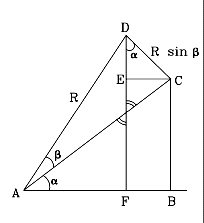Site Map Math Index Glossary Timeline Questions & Answers Lesson Plans #M-11a. Trig Proficiency drill

# (M-11)  Deriving   sin(α+β),   cos(α+β)

Given the functions (sinα, cosα, sinβ and cos β), we seek formulas that express sin(α+β) and cos(α+β). The first of these formulas is used in deriving the L4 and L5 Lagrangian points, here.

### Please verify every calculation step before proceeding!As shown in the drawing, to derive the formula we combine two right-angled triangles

ABC which has an angle α
ACD which   "   "     "      β
The long side ("hypotenuse') of ACD is AD=R. Therefore

DC = R sin β
AC = R cos β
Similarly

BC = AC sin α = R cos β sin α
AB = AC cos α = R cos β cos α
The triangle ADF is right-angled and has the angle (α+β). Therefore

R sin (α+β) = DF
R cos (α+β) = AF

### Start by deriving the sine:

R sin (α+β) = DF  =  EF + DE  =  BC + DE
Note in the drawing the two head-to-head angles marked with double lines: like all such angles, they must be equal. Each of them is one of the two sharp ("acute") angles in its own right-angled triangle. Since the sharp angles in such a triangle add up to 90 degrees, the other two sharp angles must be equal. This justifies marking the angle near D as α, as drawn in the figure.

In the right-angled triangle CED

DE = DC cos α = R sin β cos α
EC = DC sin α = R sin β sin α
Earlier it was already shown that
BC = R cos β sin α
AB = R cos β cos α
Therefore

R sin (α+β)  =  BC+DE  =  R cos β sin α + R sin β cos α

Cancelling R and rearranging α to precede β

sin (α+β)  =  cos β sin α + sin β cos α

### Similarly, for the cosine

R cos (α+β) = AF = AB –FB = AB –EC =

= R cos β cos α – R sin β sin α

Cancelling R and rearranging

cos (α+β)  =  cos α cos β – sin α sin β

 Application of these formulas:  #34b  The L4 and L5 Lagrangian Points More "Trig": M-12.   The Tangent Author and Curator:   Dr. David P. Stern      Mail to Dr.Stern:   stargaze("at" symbol)phy6.org . Last updated 9-17-2004